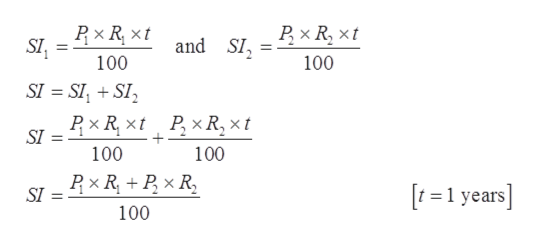# a total of \$12,000 is invested in tow funds paying 9% and 11% simple interest.  if the yearly interest is \$1180 how much of the \$12, 000 is invested at each rate.

Question
39 views

a total of \$12,000 is invested in tow funds paying 9% and 11% simple interest.  if the yearly interest is \$1180 how much of the \$12, 000 is invested at each rate.

check_circle

Step 1

Given, total invested amount, two interest rates and total yearly interest

Step 2

Let us assume, x amount invests at 9% Simple Interest and rest amount invest at 11% Simple Interest.

Step 3

So, the interest at amo...help_outlineImage Transcriptioncloseand SI SI, 100 100 SI SISI PxRxt PxR, xt SI 100 100 t=1 years] SI 100 fullscreen

### Want to see the full answer?

See Solution

#### Want to see this answer and more?

Solutions are written by subject experts who are available 24/7. Questions are typically answered within 1 hour.*

See Solution
*Response times may vary by subject and question.
Tagged in
MathAlgebra

### Other